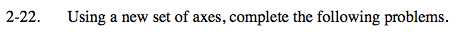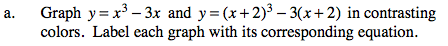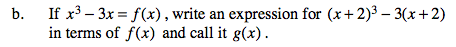### Home > PC > Chapter 2 > Lesson 2.1.2 > Problem2-22

2-22.
1. Using a new set of axes, complete the following problems. 2-22 HW eTool (Desmos) Homework Help ✎

1. Graph y = x3 − 3x and y = (x + 2)3 − 3(x + 2) in contrasting colors. Label each graph with its corresponding equation.

2. If x3 − 3x = f(x), write an expression for (x + 2)3 − 3(x + 2) in terms of f(x) and call it g(x).g(x) = f(x + 2)

Use the eTool below to view the graphs.
Click the link at right for the full version of the eTool: PCT 2-22 HW eTool